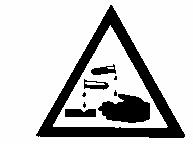# Mock exam paper B Higher Level Marking Scheme

PRE-LEAVING CERTIFICATE EXAMINATION, 2012

__________________________

ENGINEERING — HIGHER LEVEL

__________________________

Marking Scheme

__________________________Page 1 of 24

LEAVING CERTIFICATE ENGINEERING

MATERIALS AND TECHNOLOGY

(Higher Level – 300 marks)

Marking Scheme 2012

Answer Question 1, Sections A and B and Four other questions.

Question 1 Section A – 50 marks

Any ten @ 5 marks each.

1. 3 + 2
2. 5
3. 5
4. Any two @ 3 + 2
5. 3 + 2
6. 3 + 2
7. 3 + 2
8. Any two @ 3 + 2
9. 5
10. 5
11. 5
12. 5
13. 3 + 2

Question 1 Section B – 50 marks

1. 2+2+2+2+2
2. Any three at 3+3+3+1
3. three @ 3 + 1
4. (i) 5

(ii) 5

(r) 3+3+3+1

Question 2 – 50 marks

1. Any two @ 8 + 8
3. 5
4. 5
5. (i) 6
6. Describe 5 Diagram 5

Page 2 of 24

Question 3 – 50 marks

1. Any two @ 8 + 8
2. (i) 14: (7 Regions @ 2 marks each)
3. 2 + 2
4. (i) 4

(ii) 12

Question 4 – 50 marks

1. Any two @ 8 + 8
2. (i) 4
3. 2 + 2 + 2 + 2 + 2
4. 4
5. (i) 4 + 4
6. 8

Question 5 – 50 marks

1. (i) 4
2. 10
3. 4
4. Any three @ 5 + 5 + 5
5. Any one @ Describe 8 Diagram 8

OR

1. (i) 4 + 4
2. 4 + 4

Question 6 – 50 marks

1. (i) Name 2 Operation 6
2. 2 + 2 + 2
3. 2
4. Any three @ 6 + 6 + 6
5. (i) 5
6. 5
7. 3 + 3

Page 3 of 24

Question 7 – 50 marks

1. Any three @ 6 + 6 + 6
2. (i) 8

(ii) 4 + 4

(c) Any one @ 16

OR

(c) (i) 4

(ii) 4 + 4 + 4

Question 8 – 50 marks

(a) Any one @ Name 8

Application 8

(b) Any three @ 6 + 6 + 6

(c) Describe 8

Diagram 8

OR

(c) (i) Identify 2 + 2 + 2 + 2

(ii) Operation 4

Application 4

Question 1 (100 marks)

Section A – 50 marks

(a) (i) Substitutional defect

(ii) Interstitial defect3 + 2(b)

(i)

(ii)

Corrosive substance: a substance that may destroy living tissues.

Caution: warning of an immediate danger

5

1. Acetylene if directly compressed into a cylinder would explode under high pressure. Acetylene cylinders have to be packed with a porous material which is filled with acetone. Acetone is capable of absorbing up to 25 times its own volume of acetylene for each atmosphere of pressure applied to it. Dissolved acetylene is the name given to this
 form of acetylene fuel. 5

Page 4 of 24

1. (i) Casting
2. Compression moulding / Injection moulding

(iii) Compression moulding (Any two) 3 + 2

1. Metal creep is the slow defor...